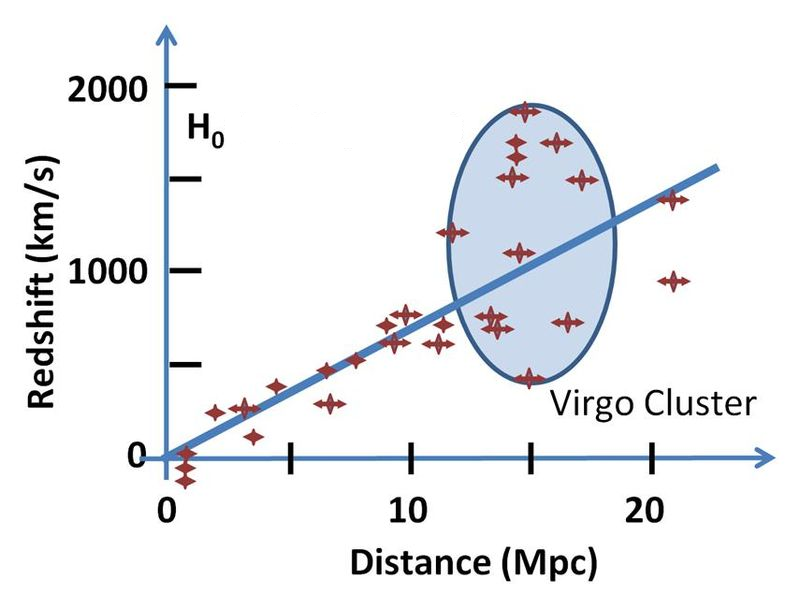# Hubble Law Distance Calculator

Created by Álvaro Díez
Reviewed by Dominik Czernia, PhD and Jack Bowater
Last updated: Jun 05, 2023

If you are wondering "What is Hubble's law?" or "What is the value of the Hubble constant?", the Hubble law distance calculator is the place to be! We will show you how to calculate the speed any galaxy is moving at, given its distance to Earth, using the Hubble's law equation.

We will also explain what redshift is, and the history and controversy behind Hubble's law definition.

## What is Hubble's Law? - Hubble's law definition

When astronomers first saw all the galaxies around us, they discovered that all of them seemed to be moving away from us. In fact, the speed at which they "run away" from us is proportional to the distance between us and them. The first people to notice this were Edwin Hubble and George Lemaitre.

Hubble's Law definition is the empirical fact that galaxies (and everything) in the universe move away from us faster the further they are from us. This proportionality was thought to be constant (now we know that it is not), and the value of this constant speed was, understandably, named after Dr. Hubble.The way to measure the speed at which galaxies move away from us is to look at their change in color or redshift. As weird as it may sound, when an object moves away from us, its color becomes slightly redder. For normal speeds, this is practically undetectable, but when we get close to the speed of light this phenomenon becomes more and more perceptible.

## What does Hubble's Law tell us?

When you look around and see that EVERYTHING is moving away from you and that it does so faster the further you are from it... there is only one possible explanation: everything moves away from everything. The fact that it seems to move away from us is because we have our own point of reference, the center of our observable universe.

Imagine a piece of fabric, on which you have marked a series of dots in permanent marker. All of the dots are at the same distance from each other. When you start stretching that fabric, all the points will be further and further away from each other. If you kept your view centered on one dot, you would see that all of the other dots are "running away" from it.

In our universe, the same thing happens. The universe is expanding, and, as a consequence, all things are getting further away from each other. The reason this is not constant, but instead slightly accelerated (as far as we can tell), is Dark energy. You can learn more about this in our universe expansion calculator (probably the best calculator about the universe we have ever made).

## How to use the Hubble law distance calculator?

The Hubble law distance calculator is very simple in principle. It is based on Hubble's law equation. As we have teased before, Hubble's law equation shows the proportionality of distance and speed for distant galaxies.

$v = H_0\times D$

where,

• $v$ — The speed of the galaxy (positive is away from us);
• $H_0$ — The Hubble constant; and
• $D$ — The distance to the galaxy.

In the calculator, you can input either the speed or the distance, and it would automatically give you the other value. In case you have already picked a side on the Hubble debate, you can hit the advanced mode and input your own preferred value for the Hubble constant.

You can also go into the Advanced mode to see the value of the Hubble constant. Feel free to edit its value to match your assumptions or to explore other scenarios.

If you are interested in calculating the distance between the earth and the stars, you can check out our parallax calculator.

## Hubble constant and "Hubble trouble"

The Hubble constant is a contentious topic right now, and researchers cannot agree on one unique value. Measuring it by looking at distant galaxies yields a value of $73.4\ \mathrm{km/s}$ per $\mathrm{Mpc}$. If you look at the remnants of the Big Bang, Hubble's constant is $67.7\ \mathrm{km/s}$ per $\mathrm{Mpc}$.

We know discrepancy is not due to experimental error, so what gives it? There must be a hole in our knowledge of the universe that we must fix! There isn't anything more frustrating, yet exciting, for physicists and space lovers alike.

In this Hubble law distance calculator, we use $70.3\ \mathrm{km/s}$ per $\mathrm{Mpc}$ (somewhere in the middle), but you can change it yourself by clicking Advanced mode.

🙋 Looking for other astrophysics calculators? Our Schwarzschild radius calculator and the redshift calculator!

Álvaro Díez
Distance
Mpcs
Speed
km/s
People also viewed…

Use our blast radius calculator to estimate non-fragment flying distance outside which no less than one fragment is expected to fly.

### Circle skirt

Circle skirt calculator makes sewing circle skirts a breeze.

### Lost socks

Socks Loss Index estimates the chance of losing a sock in the laundry.

### Spherical capacitor

Use this spherical capacitor calculator to determine the capacitance of a spherical capacitor filled with a dielectric.